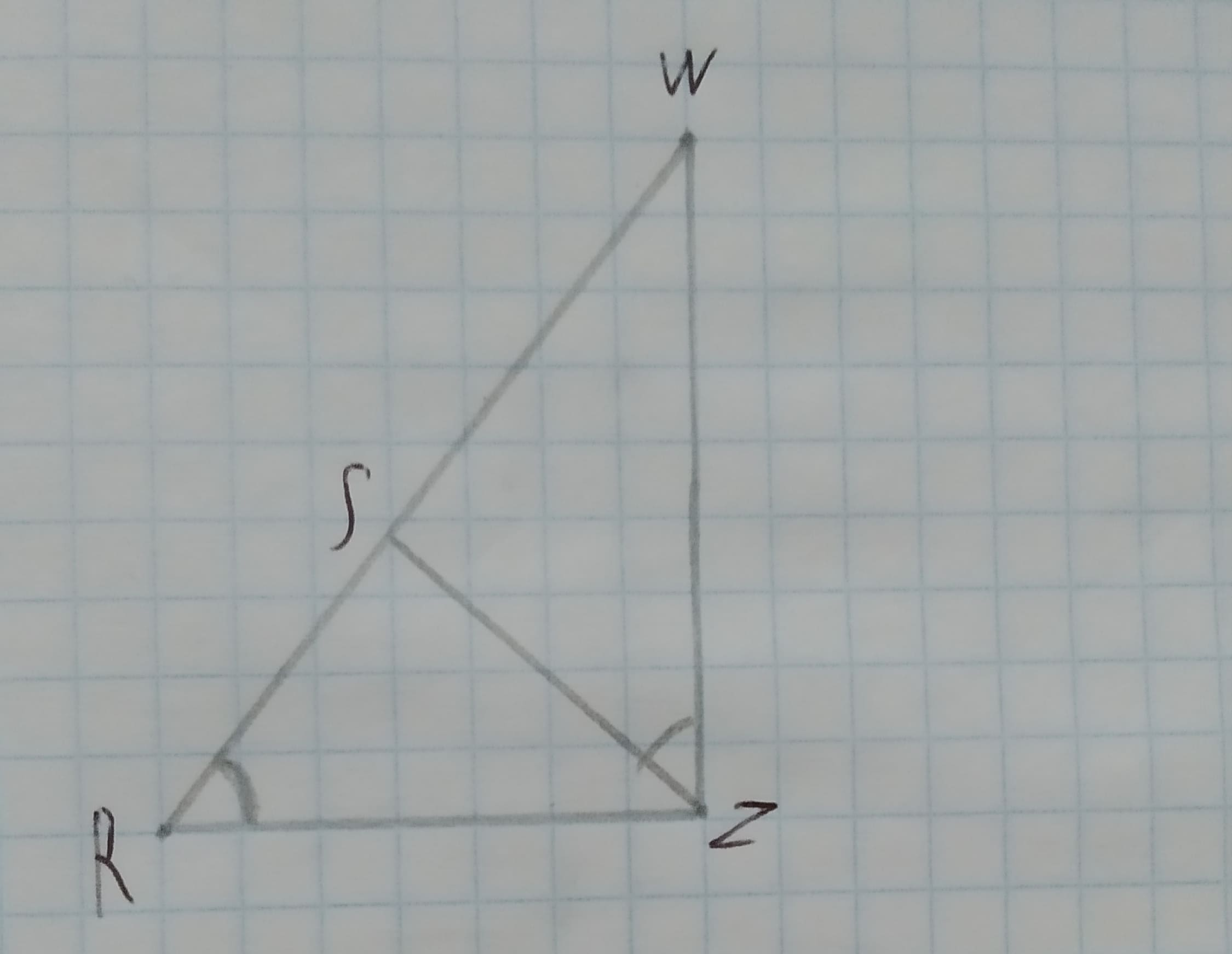# To Complete: the statement /_RWZ=? in the figure shown.Given:Figure is shown below.12210202811.jpgChardonnay Felix 2020-12-22 Answered

To Complete: the statement $\mathrm{△}RWZ=$? in the figure shown.
Given:
Figure is shown below.You can still ask an expert for help

• Questions are typically answered in as fast as 30 minutes

Solve your problem for the price of one coffee

• Math expert for every subject
• Pay only if we can solve itAlix Ortiz
Calculation:
In $\mathrm{△}RWZ\phantom{\rule{1em}{0ex}}\text{and}\phantom{\rule{1em}{0ex}}\mathrm{△}ZWS$.
$\mathrm{\angle }WRZ\stackrel{\sim }{=}\mathrm{\angle }WZS\therefore$(Given)
$\mathrm{\angle }W\stackrel{\sim }{=}\mathrm{\angle }W\therefore$(Common)
$WZ\stackrel{\sim }{=}WZ\therefore$(Common)
By AAS similarity, $\mathrm{△}RWZ\sim \mathrm{△}ZWS$.
Therefore, the answer is $\mathrm{△}ZWS$.